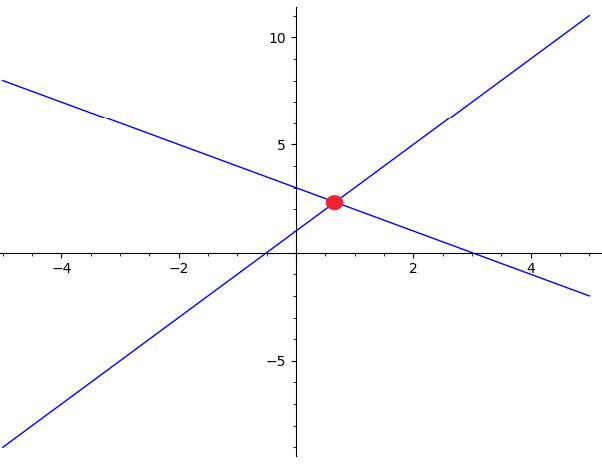# Intersection of two lines grapher

Instructions: Use this graphing tool to plot the graph of two lines that you provide. Please type two linear equations.Type a linear equation (Ex: 3x - 2y = 3 + 2/3 x, etc.)Type another linear equation (Ex: 3x - 2y = 3 + 2/3 x, etc.)(Optional) Minimum x =(Optional) Maximum x =

## More about tool to find the intersection between two lines

Lines are commonly used to represent important variables in different disciplines. For example, in Economics, the demand and supply functions are typically expressed a linear functions, and as such, their graphs will be lines simply.

And in that context of Economics supply and demand, the intersection of the lines that represent supply and demand corresponds to the market equilibrium, a pretty important concept.

Finding the intersection between two lines it the graphical equivalent to solving a system of 2x2 equations, with the formality that solving a system of equations provide an exact solution (when there is a solution), whereas graphing two lines and finding its intersection point can only be done approximately in most cases.### How do you find the intersection on a grapher?

In this case, with this graph intersection calculator, all you have to do is to provide a valid linear equation to identify the lines you want to find the intersection point for. You can write the equation in its standard form or in its slope intercept formfor each of the line.

You have flexibility in terms of how to write the equation, provided that the equations you type are valid linear equations.

### What is the formula for the point of intersection of lines?

There is no one formula to find the intersection of two lines. For starters, it could happen that the lines do not intersect each other. One robust method of solving analytically for the intersection of two lines is Cramer's method.

Ultimately, finding the intersection of two lines is the same a solving a system of equations, and there could be different strategies that are more appropriate than others, depending on the structure of the equations of the lines.